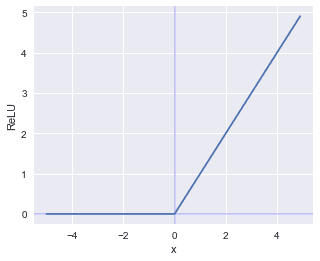search
Search
Unlock 100+ guides
search toc
close
Cancel
Post
account_circle
Profile
exit_to_app
Sign out
search
keyboard_voice
close
Searching Tips
Search for a recipe:
"Creating a table in MySQL"
Search for an API documentation: "@append"
Search for code: "!dataframe"
Apply a tag filter: "#python"
Useful Shortcuts
/ to open search panel
Esc to close search panel
to navigate between search results
d to clear all current filters
Enter to expand content previewDoc SearchCode Search BetaSORRY NOTHING FOUND!
mic
Start speaking...Voice search is only supported in Safari and Chrome.
Shrink
Navigate to
check_circle
Mark as learned
thumb_up
0
thumb_down
0
chat_bubble_outline
0
Comment
auto_stories Bi-column layout
settings

# Comprehensive Guide on ReLU

schedule Aug 11, 2023
Last updated
local_offer
Machine LearningPython
Tags
expand_more
mode_heat
Master the mathematics behind data science with 100+ top-tier guides
Start your free 7-days trial now!

Rectified linear units, or ReLU, is an activation function that is commonly used for neural networks. The mathematical formula for ReLU is quite simple:

$$f(x)=\max(0,x)$$

This formulation is actually equivalent to the following:

$$f(x)= \begin{cases} x&(x\gt0)\\ 0&(x\le0) \end{cases}$$

Graphically, ReLU would look like the following:# Implementation in Python

The implementation of ReLU is straight-forward:

 import numpy as npdef relu(x): return np.maximum(0,x) 

# Derivative of ReLU

The derivative of ReLU is straight-forward - we just need to consider the two cases:

1. when $x$ is less than or equal to zero - the derivative would simply be 0 since the slope is flat

2. when $x$ is larger than zero - the derivative would be 1 since we just have a linear curve $y=x$.

Mathematically, this is the following:

$$\frac{\partial{y}}{\partial{x}}= \begin{cases} 1&(x\gt0)\\ 0&(x\le0) \end{cases}$$
thumb_up
thumb_down
Comment
Citation
Ask a question or leave a feedback...
thumb_up
0
thumb_down
0
chat_bubble_outline
0
settings
Enjoy our search
Hit / to insta-search docs and recipes!